# Wordle is a game of chance

Many of the proposed strategies use the notions introduced by Claude Shannon to solve problems of communication…

# Wordle is a game of chance

William Casselman
University of British Columbia

The game Wordle, which is found currently on the New York Times official Wordle site, can be played by anybody with internet access. It has become extremely popular in recent times, particularly among mathematicians and programmers. One programmer comments facetiously, “At the current rate, I estimate that by the end of 2022 ninety-nine percent of all new software releases will be Wordle clones.”

The point for us is that it raises intriguing questions about the nature of information, and offers good motivation for understanding the corresponding mathematical theory introduced by Claude Shannon in the 1940s.

### How the game is played

When you visit the official Wordle web site, you will be faced with an empty grid that looks like this: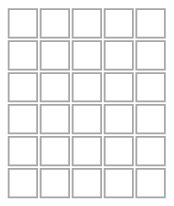Every day a secret new word of five letters is chosen by the site, and you are supposed to guess what it is by typing candidates into successive rows, responding to hints offered by the site regarding them. For example, today (May 22, 2022) I began with the word slate, and the site colored it like this: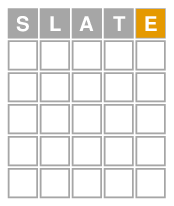What this means is that the secret word of the day has no letters “S”, “L”, “A”, or “T”, but does have a “E” in some location other than the last. I next entered the word homer and got in response:This means that the secret word does not contain at all either an “H” or an “R”; contains an “O” in the second place and an “E” in the fourth place; and contains an “M” somewhere other than the third place. With my next choice I was lucky: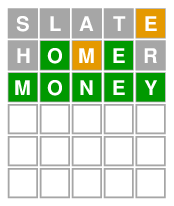This is a pretty good session—the average game is predicted to require about three and a half tries. So three is better than average, and most of the time you should get the answer in at most four.

I must now tell you the precise rules for coloring proposed answers. If neither the secret word nor your proposal has repeated letters, the rules are very simple: (1) a square is colored green if your letter and that of the secret word are the same at that location; (2) a square is colored yellow if the secret word does contain the corresponding letter somewhere, but not at this particular location; (3) the square is colored gray if the corresponding letter in your guess does not appear at all in the secret word.

But if there are repeated letters, there is some potential ambiguity that has to be dealt with. First of all, all exact coincidences are colored green, and as this is done they are removed from your guess and, internally, from the secret word. Under consideration there are now two words of length possibly less than five, for which there are no exact coincidences. The remaining guess is now scanned left to right. A location is colored yellow if it occurs somewhere in the secret word, and it is removed from the secret word. The location is colored grey if it now occurs nowhere in the secret word.

For example, if the secret word is decoy and your guess is odder, since there is only one “D” in decoy it will be colored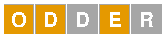Scanning left to right is an arbitrary choice, and scanning in the opposite direction would give a different coloring. One consequence of this rule and some other elementary reasoning is that some colorings, such asor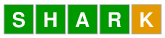can never occur.

Incidentally, the NYT Wordle site contains a convenient keyboard displayed underneath the grid, which illustrates graphically how the coloring is to be interpreted.

There are a few more somewhat arbitrary things you should know about. The principal one is that the Wordle grid will accept only a proper subset of English words of five letters, and many of the ones it does accept will probably be unfamiliar to you, such as aahed, aalii, and aarti, as well as zygal, zygon, and zymic. If you submit a word that will not be accepted, the Wordle display will complain by shuddering. The current list of words that will not cause a shudder has 12,957 words on it, and is itself divided into two subsets—the list of the approximately 2,300 words which are in fact possible answers (i.e., made up of words which should be familiar) and the rest (compiled from a huge list of all possible words in English text), which can help you to find answers even though they are not themselves among the possible answers. (You might see on the Internet mentions of 12,972 acceptable submissions. This was the original number. The game changed a bit when the NYT took it over, eliminating a few of the accepted words that might offend some people.)

### The mathematics of information

The Internet is full of advice on how best to play, but I’ll say very little about that. What is interesting to a mathematician is that many of the proposed strategies use the notions introduced by Claude Shannon to solve problems of communication and elucidate the notion of redundancy.

In fact, redundancy in English is what Wordle is all about. What do I mean by redundancy? Most succinctly, that not all sequences of five letters of the alphabet are English words. (One constraint is that the ones that do occur must be pronounceable.) In other words, certain sequences of letters cannot appear. For example, in standard English (although not in Wordle’s list of acceptable words, alas) a “q” is always followed by a “u”, so the pairs “qa”, “qb”, … are forbidden. The “u” following a “q” is therefore redundant. Another way of saying this is that “u” after “q” conveys no new information—it will not help to distinguish one word from another.

For an example more relevant to Wordle, suppose you find yourself facing the array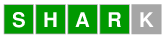Just as “u” always comes after “q”, here the last letter must be either “d”, “e”, or “p”, making possible answers shard, share, or sharp. So here the final letter carries information—it picks out one of three possibilities—but not a lot, since the possible options are very limited.

These two examples illustrate the general fact: information is the resolution of uncertainty. The more uncertainty there is, the more information is conveyed by choosing one of the options. The mathematical theory of information initiated by Shannon makes this quantitative.

To understand the relation between information and mathematics, I’ll look now at a simpler guessing game, a variant of ‘twenty questions’. In this game, somebody chooses a random number $n$ such that $0 \le n \lt 2^{3} = 8$ (i.e., in the non-inclusive range $[0, 8)$), and asks you to guess what it is. Any question you ask will be answered with only a ‘yes’ or ‘no’. How many questions might you have to ask?

The simplest strategy is to start with “Is it 0?” and continue with possibly 7 more. But this is certainly unnecessarily inefficient. The best way to phrase the optimal procedure is to express numbers in the range $[0, 8)$ in base $2$ notation. Thus $5$ is expressed as $111$, since $$5 = 1 + 0 \cdot 2 + 1 \cdot 4 .$$ The coefficients in such an expression are called bits. With this mind, you have only to ask three questions: is the $i$-th bit equal to $0$? for $i = 0$, $1$, and $2$. Whether the answer is ‘yes’ or ‘no’, you gain one bit of information.

The drawback to this procedure is that you will never get by with fewer than three questions, whereas with a naive strategy you might be lucky and get it in one. Sure, but you are more likely to be unlucky! In the naive scheme the average number of guesses is $(1 + 2 + \cdots + 7 + 8)/8 = 4.5$, whereas in the other it is $3$. When the number of choices is $2^{3}$ the difference in the average number of questions asked is small, but if $2^{3}$ is replaced by $2^{20}$ the difference is huge.

Very generally, a game with $2^{n}$ possible items may be played by asking only $n$ questions with ‘yes’ or ‘no’ answers and receiving in return $n$ bits of information. Another way to put this: a string of zeroes and ones of length $n$, chosen randomly, conveys $n$ bits of information. As a simple variant of this, suppose each item of the string is a number in the range $[0, 2^{k})$. Then a string of such numbers of length $n$ is equivalent to one of $nk$ bits, and hence conveys $nk$ bits of information.

But now Shannon posed the question: suppose the bits are not chosen randomly? Then less information will be conveyed, in general. Can we measure how much?

For example, suppose the string is of length $2n$, and may therefore be considered a string of $n$ numbers in the range $[0, 4)$. Suppose further that each $k$ is constrained to the range $[0, 3)$ (i. e. with $0 \le k \le 2$), so that in effect the string is the expression of a number in base $3$. I’ll call is a $3$-string. Instead of $4^{n}$ possible strings, there are only $3^{n}$, so that fewer than $2n$ bits of information can be conveyed. Assuming that the individual ‘digits’ are chosen randomly, how much information is conveyed by such a $3$-string?

The most fruitful answer tells what happens as $n$ becomes larger and larger. Large strings of $n$ integers in the range $[0, 3)$ can be compressed, and more efficiently compressed for large $n$. A single integer $0 \le k \le 2$ requires two bits to be expressed, one in the range $[0, 9)$ requires $4$ bits, or twice as many. But $3^{3} = 27 \lt 2^{5} = 32$, so a string of $3$ requires only $5$ bits instead of $6$. In general, if $$2^{m-1} \lt 3^{n} \lt 2^{m}$$ then more than $m-1$ bits are required to specify every $3$-string of length $n$, but fewer than $m$. We can find a formula for $n$, in fact, since extracting $n$-th roots gives us $$2^{m/n – 1/n} \lt 3 \lt 2^{m/n} .$$ Since we can write $3 = 2^{\log_{2} 3}$, this is equivalent to $${ m\over n } – { 1 \over n } \lt \log_{2} 3 \lt { m \over n } .$$ so that for large $n$ we see that $m$ is approximately $n \log_{2} 3$. What Shannon says is that a random $3$-string of length $n$ carries $n \log_{2} 3$ bits of information. Since there are $3^{n}$ such strings, if these are chosen randomly each one has probability $p = 1/3^{n}$ of occurring. Shannon’s way of putting this becomes in general the recipe:

An event of probability $p \ne 0$ carries $\log_{2} (1/p)$ bits of information.

Note that since $p \le 1$, we know that $\log_{2} \frac{1}{p} \ge 0$, so this is always non-negative. When $p = 1$ the event always takes place. There are no surprises. Sure enough, $\log_{2} 1 = 0$, so Shannon’s rule says that no information is conveyed. The rarer an event, the more surprising it is, and the more information it conveys: “Dog bites man” is nothing new, but “Man bites dog” is a different story.

Let’s look at one simple example. Suppose we are again playing ‘three questions’. You feel lucky and can’t resist blurting out, “Is the number 6?” If the answer is ‘yes’, you have acquired, as we have already seen, three bits of information. But how much information does a ‘no’ gives you? All we can say immediately is that it isn’t much, because it has only reduced the number of possibilities fom $8$ to $7$. Now if, we assume, the answers are chosen at random, the probability of getting a ‘yes’ here is $p = 1/8$, so the probability of getting a ‘no’ is $1 – p= 7/8$. Shannon assigns to it $\log_{2} 8/7 \sim 0.193$ bits of information.

### Entropy

For us, a random event is one with a finite number of outcomes. Suppose the $i$-th event to have probability $p_{i}$. If the event takes place a large number of times, what is the average amount of information seen? The $i$-th event has probability $p_{i}$, and the associated amount of information is $\log_{2} p_{i}$, so the expected average is

$$\sum_{i} p_{i} \log_{2} p_{i} .$$

Even the case $p_{i} =0$ is allowed, since

$$\lim_{p\rightarrow 0} p \cdot \log_{2} p = 0 .$$

This average is what Shannon calls the entropy of the event, measured in bits. If the event has two outcomes, with probabilities $p$ and $1-p$, the entropy is

$$(1-p)\log_{2} (1-p) + p \log_{2} p .$$

Its graph looks like this:If $p=0$ or $p=1$ there is no probability involved, and no information. The maximum possible entropy occurs when $p=1-p = 1/2$. This is when the maximum uncertainty is present, and in general entropy is a measure of overall uncertainty.

This last remark remains true when any number of outcomes are involved:

If there are $n$ outcomes, the maximum possible entropy is when all $p_{i} = 1/n$, and in this case the entropy is $\log_{2}n$.

That is to say, whenever $(p_{i})$ is any sequence of $n$ numbers $p_{i} \ge 0$ with $\sum p_{i} = 1$ then

$$\sum_{i} p_{i} \log_{2} p_{i} \le \log_{2} n .$$

This is immediately evident when $n=2$, since the graph of $y = \log x$ is concave downwards.In general it can then be derived by mathematical induction on $n$.

### Entropy and Wordle

What does this have to do with Wordle?

When you enter a candidate word into the Wordle display, the game replies by coloring your entry with one of three colors—it is giving you information about your proposal. But Wordle differs from 20 questions in that you can use this information to make your next guess. How much information is the game giving you? How can you best use this information to make your next submission?

The secret word is effectively a choice of one of the $2,309$ valid answers. Each of these presumably has equal probability. But the coloring reduces this number considerably—the true answer has to be one of those compatible with the coloring. For example, a few days ago I chose slate as my initial guess, and got a coloring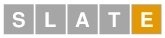I can scan through all possible answers and check which ones would give me this coloring. It happens that my choice was very bad, since there were $164$ words that do this. We can see exactly how bad this is by making a graph like the following:This graph was constructed in the following way: I scanned through all of the 2,309 possible answers and computed the coloring it would give. I used this to list for each colour all of the compatible answers. I made a list of the sizes for each color, and then sorted the list by magnitude. For example, the largest count was 221, corresponding to all grays. But the second highest was the one I got, at 164 (marked on the graph). As you can see, there was a very large reservoir of things I might have hoped for.

Could I have made a better choice of first guess? Well, what should be clear from what I write just above, each possible first guess gives me a graph like the one above. What I would like to see is a graph with a somewhat uniform height, for which the likelihood of narrowing my choices down is large. I display below a few graphs for other first guesses.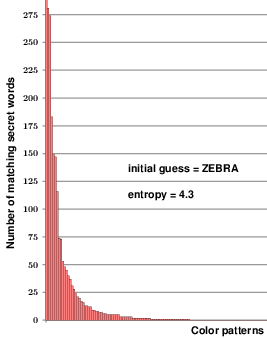It turns out that it is impossible to get a uniform height, but that some choices do much better than others. The point is that the uniformity is maximized if the entropy of a certain probability is maximized. Every choice of a starting word assigns a colour to every possible answer. These colors partition the set of possible answers, and if $n_{i}$ answers gives rise to the same color $i$ then $\sum n{i} = 2,309 = n$. I set $p_{i} = n_{i}/n$. It is the entropy of this probability distribution that is displayed in the graphs above, and you can see that a choice is better if the entropy is relatively large.

### A final remark

The idea of using entropy to find optimal ways of playing Wordle have proliferated on the Internet. Many have used entropy to make a best first guess—among these are crane, which is the one used by the official NYT advisors, and slate. Very few of these add something new, and most seem to be just taking their ideas from the video of Grant Sanderson that I mention below. (This seems to be the original investigation of entropy.)

I don’t want to add to this literature, but I do want to discuss the question of best second choices, about which less is said. It is a relatively simple calculation to list all possible answers that will colour a first guess in a given way. For example, as I have already mentioned my choice of slate above came up with 164 possibilities. This is a severe reduction of the original 2,409. But one of the quirks of Wordle is that choosing your second guess from this list might not be best. For example, if you get the coloringyou know that the secret word must be sharp, shard, or share. The obvious thing to then do is try these, one by one. However, that might take three tries, while a careful choice of some totally different word—for example pedal—will give it to you in two tries by eliminating two of the three possibilities.

Absolutely optimal strategies for Wordle are now known and posted on the Internet. But these miss the real point—I’d like to see more theoretical discussion of exactly what Wordle’s colorings tell you.

1.